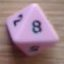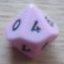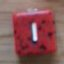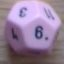How many 6s?

## How many 6s?

 Tue Jan 16 16:46:58 2018   by   Sharp I'm trying to calculated the probability of all the different ways you can make a pool of dice totalling 6 by adding values together from a larger pool. so if I rolled 6d6 I would want to count all the 6's then all the 5+1 and remove them form the pool, the 4's and 2's, remove then 4's and 2x1's etc:\ 6\ 5+1\ 4+2\ 4+1+1\ 3+3\ 3+2+1\ 3+1+1+1\ 2+2+2\ 2+2+1+1\ 2+1+1+1+1\ 1+1+1+1+1+1I have tried everything I can think of for most of the day but I keep running around in circles. I think I need to do a multiset keep. :( Thu Jan 18 09:40:33 2018   by   Torben As I understand your idea, you want to do this without replacement, so a pool of 3 3 2 1 will generate only one sum of 6, even though you can make this sum in two different ways.As far as I can see, the order in which you pick dice sets that add to six does not matter, so you might as well start with picking sixes, then fives and ones, and so on, as you suggest. I'm not sure you can do it much easier than simply trying the combinations that you list above in some order.This can be done in Troll like this:`function sixes(pool) =  if call msubset({5,1}, pool) then 1 + call sixes(pool -- {5,1})  else if call msubset({4,2}, pool) then 1 + call sixes(pool -- {4,2})  else if call msubset({4,1,1}, pool) then 1 + call sixes(pool -- {4,1,1})  else if call msubset({3,3}, pool) then 1 + call sixes(pool -- {3,3})  else if call msubset({3,2,1}, pool) then 1 + call sixes(pool -- {3,2,1})  else if call msubset({3,1,1,1}, pool) then 1 + call sixes(pool -- {3,1,1,1})  else if call msubset({2,2,2}, pool) then 1 + call sixes(pool -- {2,2,2})  else if call msubset({2,2,1,1}, pool) then 1 + call sixes(pool -- {2,2,1,1})  else if call msubset({2,1,1,1,1}, pool) then 1 + call sixes(pool -- {2,1,1,1,1})  else if call msubset({1,1,1,1,1,1}, pool) then 1 + call sixes(pool -- {1,1,1,1,1,1})  else count 6 = pool function msubset(A,B) = if A--B then {} else 1`You call this by `call sixes(pool)`, for example`p := 6d6;[p,call sixes(p)]` Thu Jan 18 16:48:30 2018   by   Sharp Thanks, I think that's really clever.